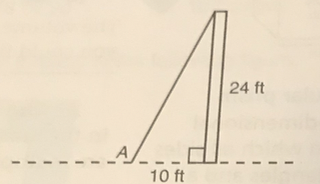## Labels

### GED Math Review: The Pythagorean Theorem

Questions:
Directions: Choose or write the answer to each question.

1. The bottom of a ladder is placed 8 feet from the wall of a house. The wall and the ground form a right angle. If the ladder is 15 feet (to the nearest foot)?• 12
• 13
• 17
• 23
2. Bill wants to find the width of the pond. He places stakes at A and B and then finds C so that C is a tight angle. This makes ∆ABC a right triangle. If AC = 60 feet and BC = 80 feet, about how far is it from A to B?• from 85 to 95 ft
• from 95 to 105 ft
• from 105 to 115 ft
• more than 115 ft
3. Jan builds a platform with a top in the shape of a trapezoid. The plans call for a diagonal brace to be added. What is the length of the brace to the nearest inch?• 64
• 88
• 96
• Not enough information is given.
4. The pole is perpendicular to the ground. A wire is stretched from the ground to the top of the pole. If point A is moved 8 feet farther from the pole, how much more will be needed to reach the top of the pole?• 4 ft
• 6 ft
• 26 ft
• 30 ft
5. The lengths of the sides of a triangle are 1, 1 1/3, and 1 2/3. Is the triangle a right triangle? Circle the answer that completes the sentence.
• The triangle is a right triangle.
• The triangle is not a right triangle.

1. 13
2. from 95 to 105 ft
3. 96
4. 4 ft
5. The triangle is a right triangle.

Reference:

Complete Test Preparation for the GED Test 2014 by Steck-Vaughn